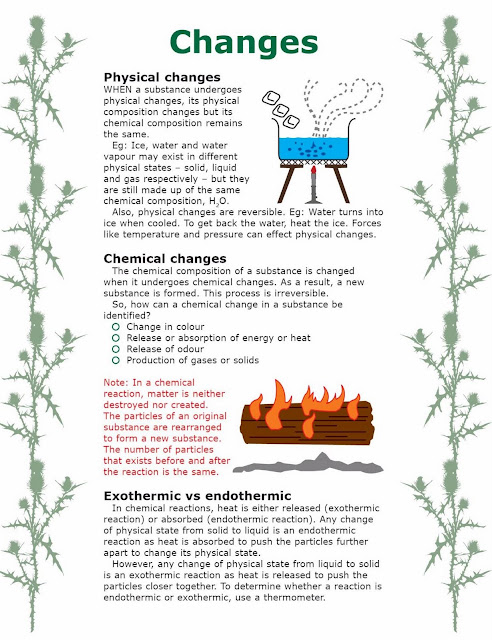## Saturday, 24 September 2016

### Chapter 7 ThermoChemistry

#### Inter part 1 chemistry ThermoChemistry

Q.1 Which of the following statements is contrary to the first law of thermodynamics?(a) energy can neither be created nor destroyed
(b) one form of energy can be transferred into an equivalent amount of other kinds of energy
(c)  in an adiabatic process, the work done is independent of its path(d) continuous production of mechanical work with out equivalent amount of heat is possible
Q.2 The change in heat energy of a chemical reaction at constant temperature and pressure is called
(a) enthalpy change (b) bond energy
(c) heat of sublimation (d) internal energy change
Q.3 For the reaction NaOH + HCl = NaCl + H2O, the change in enthalpy is called as:
(a) heat of reaction (b) heat of formation
(c) heat of neutralization (d) heat of combustion
Q.4 Calorie is equivalent to
(a) 0.4184 J (b) 41.84 J
(c) 4.184 J (d) 418.4 J
Q.5 For a given process, the heat change at pressure (qp) and constant volume (qv) are related to each other as
(a) qp = qv (b) qp < qv
(c) qp > qv (d) qp =
Q.6 The net heat change in a chemical reaction is same whether. It is brought about in two or more different ways in one or several steps. It is known as
(a) Henry’s law (b) Joule’s principle
(c) Hess’s law (d) Law of conservation of energy
Q.7 Enthalpy of neutralisation of all the strong acids and strong bases has the same value because
(a)  neutralisation leads to the formation of salt and H2O
(b) strong acid and bases are ionic substances
(c)  acids always give rise to H+ ions and bases always furnish OH– ions (d) the net chemical change involve the combination of H+ and OH– ions to form water
Q.8 If an endothermic reaction is allowed to take place very rapidly in the air. The temperature of the surrounding air (a) remains constant (b) increase
Q.9 In endothermic reactions, the heat content of the
(a)  products is more than that of reactants
(b) reactants is more than that of products
(c)  both (a) and (b)
Q.10 Hess’s law is also called
(a)  first law of thermodynamics
(b) second law of thermodynamics
(c)  first law of thermochemistry
(d) second law of thermochemistry
Q.11 Pressure – volume work is
(a) P  v (b) F x d
(c) h  v (d) H + p v
Q.12 Kinetic energy of molecules is due to
(a) rotational energy (b) vibrational energy
(c) translational energy (d) all of these
Q.13 The condition for standard enthalpy change is
(a) 1 atm 30oC (b) 1 atm 0oC
(c) 1 atm 25oC (d) 760 atm 25oC
Q.14 The unit of enthalpy change is
(a) calorie (b) joule
(c) volt (d) coulomb
Q.15 The sum of all kinds of a system is ions or molecules of a system is
(a) vibrational energy (b) potential energy
(c) kinetic energy (d) internal energy
Q.16 An endothermic reaction is one is which
(a)  enthalpy of reactants and products are same
(b) enthalpy of products is greater than reactant
(c)  enthalpy of products is lesser than reactants
(d) heat is evolved from system
Q.17 Bomb calorimeter is used to determine
(a)  enthalpy of solution
(b) enthalpy of atomization
(c)  enthalpy of combustion
(d) enthalpy of neutralization
Q.18 Glass calorimeter is used to determine
(a)  enthalpy of combustion
(b) enthalpy of reaction
(c)  pressure–volume work
(d) none of above
Q.19 Born–Haber cycle is used to calculate
(a)  enthalpy of combustion
(b) lattice energy of ionic camps
(c)  both a and b
(d) none of above
Q.20 Born–Haber cycle is an application of
(a)  first law of thermodynamics
(b) second law of thermodynamics
(c)  first law of thermochemistry
(d) Hess’s law
Q.21 An exothermic reaction is one in which
(a)  enthalpy of reactants and products are same
(b) heat is absorbed by system
(c)  enthalpy of products is greater than reactants
(d) enthalpy of reactants is lesser than products
Q.22 A substance under observation during an experiment
(a) surrounding (b) system
(c) state function (d) universe
Q.23 Enthalpy of neutralization is merely
(a) heat of solution (b) heat of atomization
(c)  heat of combustion
(d) heat of formation of H2O
Q.24 Lattice energy of NaCl is
(a) + 500 kJ (b) – 344 kJ
(c) – 776 kJ (d) – 411 kJ
Q.25 Standard enthalpy of Al2O3 cannot be measured because
(a)  it does not catch fire
(b) it reacts with CO2
(c)  protective layer of oxide cover the surface
(d) none of aboveChanges
Q.26 Ammonium chloride dissolve in water this process is
(a) endothermic process (b) exothermic process
(c) simple hydration (d) none of above
Q.27 First law of thermodynamics is represented as
(a) delta E = q + R T (b) delta E = q + delta P
(c) delta E = q + delta P (d) E = q + w
Q.28 Pumping of water uphill is
(a) spontaneous reaction (b) exothermic reaction
(c)  non–spontaneous reaction
(d) endothermic
Q.29 In exothermic reaction delta H is
(a) positive (b) negative

(c) zero (d) none of above